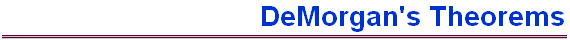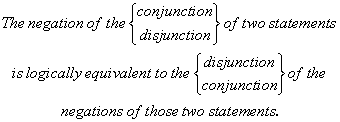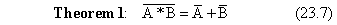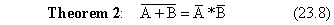There are two final theorems with which we must acquaint ourselves in order to complete our introduction to logic. These theorems are collectively called DeMorgans Theorems (or DeMorgans Laws) after the English mathematician and logician (and contemporary of George Boole) Augustus DeMorgan (1806 - 1871).  These theorems can be stated in words as follows:In the sentence above, the top word in one bracket should be read with the top word in the other; the same for the bottom words.  (This just keeps us from having to write the sentence  twice.) More elegantly and certainly more concisely, these two theorems can be stated symbolically as follows:andDeMorgans theorems were very useful in the early development of digital electronics in that a consequence of these theorems is that all logical operations (even those which we have not  discussed in this class, such as NAND, NOR, XOR, XNOR, etc.) can be reduced to combinations of NOT and AND functions or combinations of NOT and OR functions.  This means that, with only the three functions we have defined and discussed (AND, OR, and NOT), we can work out every type of logic operation! DeMorgans first theorem above will be proved in the next example.  You will prove the second theorem (possibly using the results of the first theorem) in the homework.